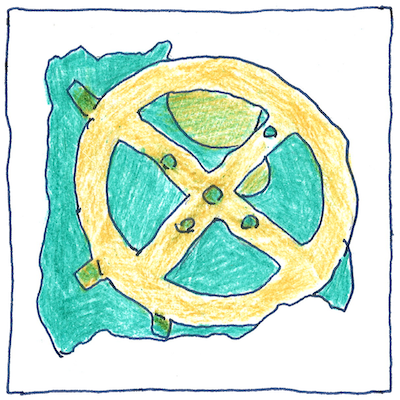# 2400 BCE—1820 CE

Tom Sharp

## Computing devices

• Sumerian abacus
• invented in Babylonia around 2400 BCE
• a positional sexagesimal table
• for placing pebbles as counters
• Chinese abacus or suanpan
• around 200 BCE
• a frame with beads sliding on rods
• five below the beam and two above
• capable of bi-quinary and hexadecimal calculations
• Roman reckoning board
• a ten-by-ten grid of holes
• holding a single count using a peg
• Roman abacus
• a bi-quinary coded-decimal place-value tray
• with upper and lower grooved columns
• for placing counters known as calculi
• *
• Apollonius of Perga’s astrolabe
• around 220 BCE and later astrolabes
• in the Byzantine and medieval Islamic world
• could calculate the time of day
• based on the stars and planets
• based on the time of day.
• The Antikythera mechanism
• around 205 BCE
• with at least thirty gears
• was based on the Egyptian calendar
• and could calculate eclipses
• positions of the sun and moon
• and phases of the moon
• A water-powered celestial globe
• by Liang Lingzan and Yi Xing in the 720s
• computed dawn, dusk,
• and phases of the moon
• A geared mechanical astrolabe
• by Abū Rayhān al-Bīrūnī in 996
• had eight gears, and his planisphere,
• a star-chart, could show the stars
• for any date and time,
• The Equatorium
• by Abū Ishāq Ibrāhīm al-Zarqālī in 1015
• computed the positions of the planets
• The Torquetum
• by Jabir ibn Aflah (Geber) in 1100
• converted horizonal, equatorial, and ecliptic
• measurements
• Ismail al-Jazari, who wrote
• The Book of Knowledge of Ingenious Mechanical Devices,
• built a large waterpowered castle clock in 1206
• that displayed the zodiac,
• orbits of the sun and moon,
• mannequins popping out of doors on the hours,
• automaton musicians that played music,
• and it was programmable in that
• seasonal length of days could be adjusted
• The Plate of Conjunctions
• by Jamshīd al-Kāshī in 1400
• calculated the times of planetary conjunctions
• *
• Napier’s bones
• made by John Napier in 1617
• to help him calculate tables of logarithms
• a set of multiplication tables
• on square-ended sticks
• that can be arranged on a board
• to multiply, divide, or find square roots
• William Oughtred
• put together two circular logarithmic rules in 1622
• to create the first slide rule
• capable of direct multiplication and division
• *
• The machine arithmétique or Pascaline
• by Blaise Pascal in 1642
• and multiply or divide
• by repeated addition or subtraction
• A non-decimal “Multiplying Instrument”
• by Samuel Morland in 1666
• added English pounds, shillings, and pence
• The Stepped Reckoner
• by Gottfried Wilhelm Leibniz in 1672
• added, subtracted, multiplied, and divided
• eight- to sixteen-digit decimal numbers
• and featured a stepped drum
• now called a “Leibniz wheel”
• The first pinwheel calculator
• by Giovanni Poleni in 1709
• such as the Odhner Arithmometer in 1873
• The Arithmometer
• by Charles Xavier Thomas in 1820
• used a Leibniz wheel and was the first
• commercially successful mechanical calculator,
• as though commercial success
• were the goal of history

## Suanpan

• on each rod to count in tens.
• to count in sixteens for units of weight.
• Cultivate thoughtlessness
• to perform calculations with minimal thought.

## Antikythera mechanism

• It used the Sothic Egyptian calendar
• and predicted the Olympic-game cycles,
• the positions of sun and moon,
• twenty-seven eclipses of the sun,
• and thirty-eight eclipses of the moon,
• various calendar cycles,
• and possibly positions
• of the five known planets.
• Egyptian names for thirteen months
• are transcribed in the Greek alphabet.
• Corinthian names for twelve lunar months
• are on the perimeter of the Metonic dial.
• It shows the path of the ecliptic
• through the twelve houses of the zodiac
• labeled by their Greek names
• and keyed to specific stars.
• It computes the Metonic cycle,
• the Saros cycle,
• the Callippic cycle,
• and the Exeligmos cycle.
• It tracked the precession of the elliptic.
• It used epicyclic gearing to track
• the eliptical orbit of the moon.
• It used a differential gear
• to track the phases of the moon.
• Nevertheless, its conception
• was finer than its manufacture,
• so that the subtleties it was designed to show
• would have been swamped
• by the crudeness of its gearing.

## Napier’s bones

• Here we have a set
• of multiplication tables
• arrangeable on a board
• to calculate products
• and quotients of large numbers.
• They are like toys
• but without a childish stigma,
• and division to subtraction.

## Slide rule

• The modern Acu-Math No. 500
• has nine linear logarithmic scales,
• three scales on each part,
• and three parts, the center free to slide.
• The alignment of scales is assisted
• by a sliding transparent hairline cursor.
• It multiplies and divides,
• finds logarithms and exponentials,
• finds squares and square roots,
• cubes and cube roots,
• finds sines and cosines,
• tangents and cotangents,
• does unit conversions
• and conversion to natural logarithms.
• The modern Acu-Math No. 500
• introduced in 1960, is obsolete.